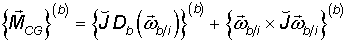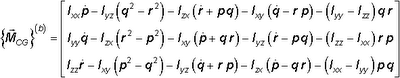## Monday, September 07, 2009

### Vector vs Scalar Expressions

Consider the well known Euler’s equations expressed in the body fixed frame:In this vector form, there are a total of five operations (one derivative, two multiplications, one cross product and one addition)

If we choose to use scalar equations:Here we have 30 multiplications, 21 subtractions and 3 additions, which add up to 54 operations. As you see, expressing equations in vector form yields 10 times less complication. It is easier to review, find errors and code vector equations. You only need code for vector/matrix multiplication, addition and cross products (they can be easily found on the internet).